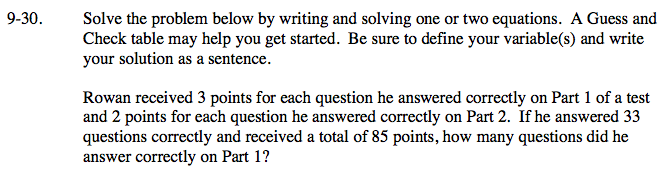### Home > AC > Chapter 9 > Lesson 9.2.1 > Problem9-30

9-30.Write a set of equations representing the problem.

Solve the second equation for y.

Substitute 33 − x in for y.

Solve for x.

Substitute 19 into one of the original equations for x.

Solve for y.

x = # of correct answers on Part 1
y= # of correct answers on Part 2

x + y = 33

3x + 2y = 85

y = 33 − x

3x + 2(33 − x) = 85

3x + 66 − 2x = 85

x = 19

19 + y = 33

y = 14